## The Particle in a 1D Box

As a simple example, we will solve the 1D Particle in a Box problem. That is a particle confined to a region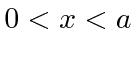. We can do this with the (unphysical) potential which is zero with in those limits andoutside the limits.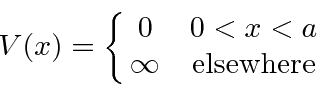Because of the infinite potential, this problem has very unusual boundary conditions. (Normally we will require continuity of the wave function and its first derivative.) The wave function must be zero atand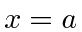since it must be continuous and it is zero in the region of infinite potential. The first derivative does not need to be continuous at the boundary (unlike other problems), because of the infinite discontinuity in the potential.

The time independent Schrödinger equation (also called the energy eigenvalue equation) iswith the Hamiltonian (inside the box)Our solutions will haveoutside the box.

The solution inside the box could be written aswhere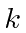can be positive or negative. We do need to choose linear combinations that satisfy the boundary condition that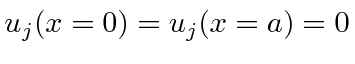.

We can do this easily by choosingwhich automatically satisfies the BC at 0. To satisfy the BC atwe need the argument of sine to be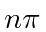there.Plugging this back into the Schrödinger equation, we getThere will only be a solution which satisfies the BC for a quantized set of energies.We have solutions to the Schrödinger equation that satisfy the boundary conditions. Now we need to set the constant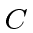to normalize them to 1.Remember that the average value ofis one half (over half periods). So we setgiving us the eigenfunctionsThe first four eigenfunctions are graphed below. The ground state has the least curvature and the fewest zeros of the wavefunction.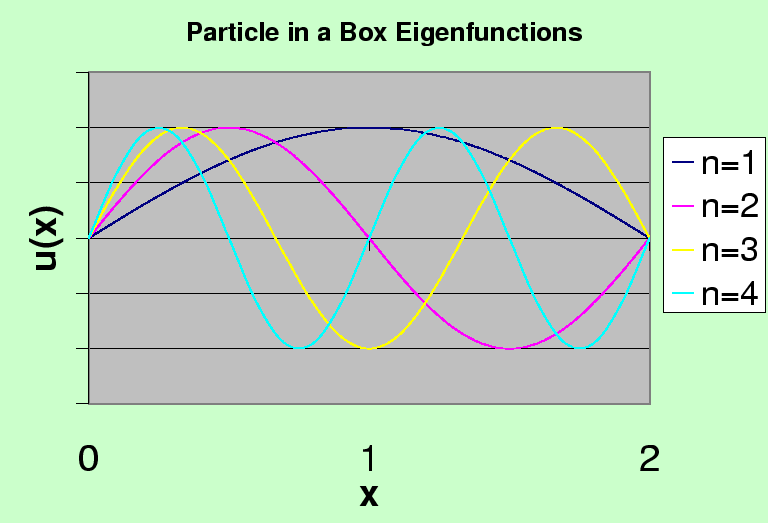Note that these states would have a definite parity ifwere at the center of the box.

The expansion of an arbitrary wave function in these eigenfunctions is essentially our original Fourier Series. This is a good example of the energy eigenfunctions being orthogonal and covering the space.

Subsections
Jim Branson 2013-04-22